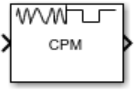# CPM Demodulator Baseband

Demodulate signal using CPM method and Viterbi algorithm

•Libraries:
Communications Toolbox / Modulation / Digital Baseband Modulation / CPM

## Description

The CPM Demodulator Baseband block demodulates an input signal that was modulated using the continuous phase modulation (CPM) method.

CPM is a modulation method with memory. The block processing includes a correlator followed by a maximum-likelihood sequence detector (MLSD) that searches the paths through the state trellis for the minimum Euclidean distance path. The block uses the Viterbi algorithm to perform MLSD.

## Ports

### Input

expand all

Input signal, specified as a scalar or column vector. The length of the input signal must be an integer multiple of the number of samples per symbol specified in the Samples per symbol parameter. For more information, see Integer-Valued and Binary-Valued Output Signals.

Data Types: `double` | `single`

### Output

expand all

Output signal, returned as a scalar or column vector. For more information, see Integer-Valued and Binary-Valued Output Signals.

#### Supported Data Types

• Double-precision floating point

• Boolean (when Output type is set to `Bit`)

• 8-, 16-, and 32-bit signed integers (when Output type is set to `Integer`)

Data Types: `double` | `Boolean` | `int8` | `int16` | `int32`

For more information on the processing rates, see Single-Rate Processing, and Multirate Processing.

## Parameters

expand all

Modulation order indicating the alphabet size, specified as a positive integer that is a nonzero power of two. M must have the form 2K for some positive integer K, where K is the number of bits per symbol.

Determines whether the output consists of integers or groups of bits, specified as `Integer` or `Bit`.

Bit mapping, specified as `Binary` or `Gray`.

• Set this parameter to `Binary` to map symbols using natural binary-coded ordering.

• Set this parameter to `Gray` to map symbols using Gray-coded ordering.

#### Dependencies

To enable this parameter, set Output type to `Bit`.

Modulation index {hi}, specified as a nonnegative scalar or column vector.

{h} represents a sequence of modulation indices. For more information, see CPM Demodulation.

Type of pulse shaping used to smooth the phase transitions of the modulated signal, specified as `Rectangular`, `Raised Cosine`, `Spectral Raised Cosine`, `Gaussian`, or `Tamed FM`. For more information on the filtering options, see Pulse Shape Filtering.

Main lobe duration of the largest lobe in the spectral raised cosine pulse, specified as a positive integer representing the number of symbol intervals used by the demodulator to pulse-shape the modulated signal.

#### Dependencies

To enable this parameter, set Frequency pulse shape to `Spectral Raised Cosine`.

Rolloff factor of the spectral raised cosine pulse, specified as a scalar in the range [0, 1].

#### Dependencies

To enable this parameter, set Frequency pulse shape to `Spectral Raised Cosine`.

Product of the bandwidth and symbol time of the Gaussian pulse shape, specified as a positive scalar. Use BT product to reduce the bandwidth, at the expense of increased intersymbol interference.

#### Dependencies

To enable this parameter, set Frequency pulse shape to `Gaussian`.

Frequency pulse shape length, specified as a positive scalar. For more information on the frequency pulse length, refer to LT in Pulse Shape Filtering.

Data symbols used before the start of simulation, specified as scalar or vector with odd integer elements in the range [– (M – 1), (M – 1)]. M represents the modulation order, which is specified by the M-ary number parameter. The Symbol prehistory parameter defines the data symbols used by the modulator prior to the first call of the block, in reverse chronological order.

• A scalar value expands to a vector of length LP – 1. LP represents the pulse length, which is specified by the Pulse length (symbol intervals) parameter.

• For a vector, the length must be LP – 1.

Initial phase offset in radians of the modulated waveform, specified as a scalar.

Symbol sampling rate, specified as a positive scalar. This parameter represents the number of samples output for each integer or binary word input. For all nonbinary schemes, as defined by the pulse shapes, this value must be greater than 1.

Block processing rate, specified as one of these options:

• `Enforce single-rate processing` — The input and output signals have the same port sample time. The block implements the rate change by making a size change at the output when compared to the input. The output width is the number of symbols (which is given by dividing the input length by the Samples per symbol parameter value when the Output type parameter is set to `Integer`).

• `Allow multirate processing` — The input and output signals have different port sample times. The output period is the same as the symbol period and equals the product of the input period and the Samples per symbol parameter value.

Traceback depth for the Viterbi algorithm, specified as a positive integer representing the number of trellis branches that the Viterbi algorithm uses to construct each traceback path. The value of this parameter is also the output delay and the number of zero symbols that precede the first meaningful demodulated symbol in the output. For more information, see Traceback Depth and Output Delays.

Output data type, specified as `double`, `boolean`, `int8`, `int16`, or `int32`. For more information, see Supported Data Types in `Out`.

## Block Characteristics

 Data Types `Boolean` | `double` | `integer` | `single` Multidimensional Signals `no` Variable-Size Signals `no`